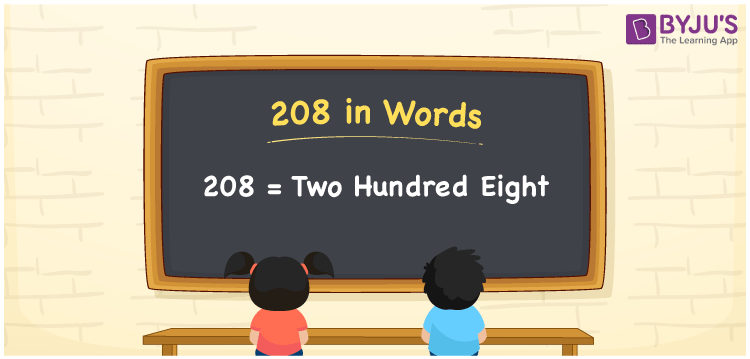# 208 in Words

208 in words is Two hundred eight. Usually, the numbers can be converted using words using the place values of the numbers. For example, 208 has 3 digits. Thus, the ones, tens and hundreds places of a number are used to write the number name 208. In this article, we are going to learn the procedure of writing the number 208 in words using the place value system in detail.

 208 in Words: Two Hundred Eight. Two Hundred Eight in Numerical Form: 208.

## 208 in English Words## How to Write 208 in Words?

The below table depicts the place values of the number 208:

 Hundreds Tens Ones 2 0 8

The expanded form of 208 is as follows:

= 2 × Hundred + 0 × Ten + 8 × One

= 2 × 100 + 0 × 10 + 8 × 1

= 200 + 8

= 208

= Two hundred eight

Hence, 208 in words is two hundred eight.

208 in words – Two hundred eight

Is 208 an odd number? – No

Is 208 an even number? – Yes

Is 208 a perfect square number? – No

Is 208 a perfect cube number? – No

Is 208 a prime number? – No

Is 208 a composite number? – Yes

## Frequently Asked Questions on 208 in Words

Q1

### Write 208 in words.

208 in words is two hundred eight.

Q2

### Simplify 200 + 8, and express it in words.

Simplifying 200 + 8, we get 208. Hence, 208 in words is two hundred eight.

Q3

### How to write two hundred eight in numbers?

Two hundred eight in numbers is 208.